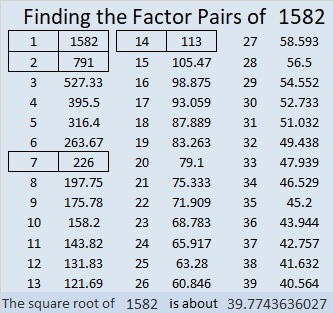# 1582 Half the Time You’ll Be Thinking about Multiples instead of Factors

Contents

### Today’s Puzzle:

In the level 6 puzzle, the possible common factors of 40 and 20 are 4, 5, and 10 while the possible common factors of 24 and 12 are 3, 4, and 6.

Don’t worry about which common factor to choose to start the puzzle. Instead, think about multiples. One of the numbers from 1 to 10 will only be able to go in one place in the top row because that’s the only place one of its multiples is in the column below it. That same number will also have only one place it can go in the first column.

You will likely look for lone multiples of particular numbers a total of five times as you solve this puzzle. Good luck!### Factors of 1582:

• 1582 is a composite number.
• Prime factorization: 1582 = 2 × 7 × 113.
• 1582 has no exponents greater than 1 in its prime factorization, so √1582 cannot be simplified.
• The exponents in the prime factorization are 1, 1, and 1. Adding one to each exponent and multiplying we get (1 + 1)(1 + 1)(1 + 1) = 2 × 2 × 2 = 8. Therefore 1582 has exactly 8 factors.
• The factors of 1582 are outlined with their factor pair partners in the graphic below.### More about the Number 1582:

1582 is the hypotenuse of a Pythagorean triple:
210-1568-1582, which is 14 times (15-112-113).

1582 is the sum of the four numbers from 394 to 397.

1582 is the sum of the seven numbers from 223 to 229.

1582 is the sum of the twenty-eight numbers from 43 to 70.

This site uses Akismet to reduce spam. Learn how your comment data is processed.